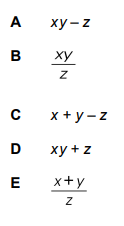# IMAT 2017 Q57 [Logarithm Expression]

Given that

log_{10} ^7 = x
log_{10}^2 = y
log_{10}^3 = z

What is log_{10}(\frac{14}{3}) expressed in terms of x, y, and z ?There are some rules for the logarithm that need to be remembered. These rules are:

1. \log _{a}x\cdot y = \log _{a}x + \log _{a}y
2. \log _{a}\frac{x}{y} = \log _{a}x - \log _{a}y
3. \log _{a} x^{m} = m \log _{a}x
4. \log _{a} \sqrt[n]{x} = \frac{1}{n} \log _{a}x

So in order to solve the question, we will use these rules. In this particular situation we will use the rules 1 and 2.

\log _{10}\frac{14}{3} = \log _{10}14 - \log _{10}3 (using rule 2) \to \log _{10}(7\cdot4) - \log _{10}3 = \log _{10}7 + \log _{10}4 - \log _{10}3 (using rule 1) \to \log _{10}7 + \log _{10}4 - \log _{10}3 = x + y - z
Which finally gives us the solution to the question.